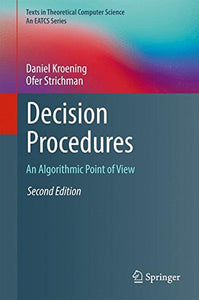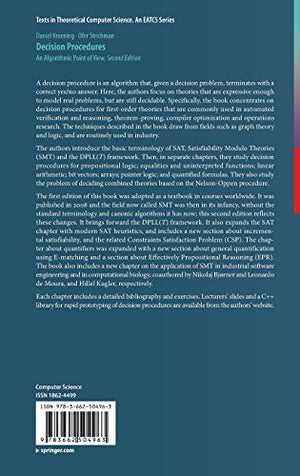# Decision Procedures: An Algorithmic Point Of View (Texts In Theoretical Computer Science. An Eatcs Series)

• Publish Date: 2016-11-23
• Binding: Hardcover
• Author: Daniel Kroening;Ofer Strichman
Regular price \$102.30 Sale price \$84.94

Attention: For textbook, access codes and supplements are not guaranteed with used items.

A decision procedure is an algorithm that, given a decision problem, terminates with a correct yes/no answer. Here, the authors focus on theories that are expressive enough to model real problems, but are still decidable. Specifically, the book concentrates on decision procedures for first-order theories that are commonly used in automated verification and reasoning, theorem-proving, compiler optimization and operations research. The techniques described in the book draw from fields such as graph theory and logic, and are routinely used in industry. The authors introduce the basic terminology of satisfiability modulo theories and then, in separate chapters, study decision procedures for each of the following theories: propositional logic; equalities and uninterpreted functions; linear arithmetic; bit vectors; arrays; pointer logic; and quantified formulas.